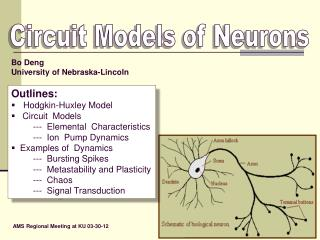# Circuit Models of Neurons - PowerPoint PPT PresentationDownload PresentationCircuit Models of Neurons

Circuit Models of NeuronsDownload Presentation## Circuit Models of Neurons

- - - - - - - - - - - - - - - - - - - - - - - - - - - E N D - - - - - - - - - - - - - - - - - - - - - - - - - - -
##### Presentation Transcript

1. Circuit Models of Neurons Bo Deng University of Nebraska-Lincoln • Outlines: • Hodgkin-Huxley Model • Circuit Models • --- Elemental Characteristics • --- Ion Pump Dynamics • Examples of Dynamics • --- Bursting Spikes • --- Metastability and Plasticity • --- Chaos • --- Signal Transduction AMS Regional Meeting at KU 03-30-12

2. Hodgkin-Huxley Model (1952) • Pros: • The first system-wide model for excitable membranes. • Mimics experimental data. • Part of a Nobel Prize work. • Fueled the theoretical neurosciences for the last • 60 years and counting.

3. Hodgkin-Huxley Model (1952) • Pros: • The first system-wide model for excitable membranes. • Mimics experimental data. • Part of a Nobel Prize work. • Fueled the theoretical neurosciences for the last • 60 years and counting. • Cons: • It is not entirely mechanistic but phenomenological. • Different, ad hoc, models can mimic the same data. • It is ugly. • Fueled the theoretical neurosciences for the last • 60 years and counting.

4. Hodgkin-Huxley Model --- Passive vs. Active Channels

5. Hodgkin-Huxley Model

6. Hodgkin-Huxley Model

7. Hodgkin-Huxley Model

8. C -I (t) The only mechanistic part ( by Kirchhoff’s Current Law)

9. Hodgkin-Huxley Model --- A Useful Clue

10. H-H TypeModels for Excitable Membranes • Morris, C. and H. Lecar, • Voltage oscillations in the barnacle giant muscle fiber, • Biophysical J., 35(1981), pp.193--213. • Hindmarsh, J.L. and R.M. Rose, • A model of neuronal bursting using three coupled first order differential • equations, • Proc. R. Soc. Lond. B. 221(1984), pp.87--102. • Chay, T.R., Y.S. Fan, and Y.S. Lee • Bursting, spiking, chaos, fractals, and universality in biological • rhythms, Int. J. Bif. & Chaos, 5(1995), pp.595--635.

11. Our Circuit Models • Elemental Characteristics -- Resistor

12. Our Circuit Models • Elemental Characteristics -- Diffusor

13. Our Circuit Models • Elemental Characteristics -- Ion Pump

14. Dynamics of Ion Pump as Battery Charger

15. Equivalent IV-Characteristics --- for parallel channels Passive sodium current can be explicitly expressed as

16. Equivalent IV-Characteristics --- for serial channels Passive potassium current can be implicitly expressed as A standard circuit technique to represent the hysteresis is to turn it into a singularly perturbed equation 0

17. Equations for Ion Pump • By Ion Pump Characteristics • with substitution and assumption • to get

18. VK =hK(IK,p) I Na =fNa(VC–ENa)

19. Examples of Dynamics --- Bursting Spikes --- Chaotic Shilnikov Attractor --- Metastability& Plasticity --- Signal Transduction Geometric Method of Singular Perturbation • Small Parameters: • 0 < e<< 1 with ideal • hysteresis at e = 0 • both C and lhave • independent time scales

20. Bursting Spikes C = 0.005

21. Neural Chaos gNa = 1 dNa = - 1.22 v1 = - 0.8 v2 = - 0.1 ENa = 0.6 • C = 0.5 • = 0.05 • g = 0.18 • = 0.0005 • Iin = 0 C = 0.005 gK = 0.1515 dK = -0.1382 i1 = 0.14 i2 = 0.52 EK = - 0.7 C = 0.5

22. Griffith et. al. 2009

23. Metastability and Plasticity • Terminology: • A transient state which behaves like a steady state is • referred to as metastable. • A system which can switch from one metastable state • to another metastable state is referred to as plastic.

24. Metastability and Plasticity

25. Metastability and Plasticity

26. Metastability and Plasticity • All plastic and metastable states are lost with only one • ion pump. I.e. when ANa= 0 or AK= 0 we have either • Is= IA or Is= -IA and the two ion pump equations are • reduced to one equation, leaving the phase space one • dimension short for the coexistence of multispike burst • or periodic orbit attractors. • With two ion pumps, all neuronal dynamics run on • transients, which represents a paradigm shift from basing • neuronal dynamics on asymptotic properties, which can • be a pathological trap for normal physiological functions.

27. Saltatory Conduction along MyelinatedAxon with Multiple Nodes Inside the cell Outside the cell Joint work with undergraduate and graduate students: Suzan Coons, Noah Weis, Adrienne Amador, Tyler Takeshita, Brittney Hinds, and Kaelly Simpson

28. Coupled Equations for Neighboring Nodes • Couple the nodes by adding a linear resistor between them Current between the nodes

29. The General Case for N Nodes • This is the general equation for the nth node • In and out currents are derived in a similar manner:

30. C=.1 pF C=.7 pF (x10 pF)

31. Transmission Speed C=.1 pF C=.01 pF

32. Closing Remarks: • The circuit models can be further improved by dropping the • serial connectivity assumption of the passive electrical • and diffusive currents. • Existence of chaotic attractors can be rigorously proved, • including junction-fold, Shilnikov, and canard attractors. • Can be easily fitted to experimental data. • Can be used to build real circuits. • Kandel, E.R., J.H. Schwartz, and T.M. Jessell • Principles of Neural Science, 3rd ed., Elsevier, 1991. • Zigmond, M.J., F.E. Bloom, S.C. Landis, J.L. Roberts, and L.R. Squire • Fundamental Neuroscience, Academic Press, 1999. • References: • [BD] A Conceptual Circuit Model of Neuron, Journal of Integrative Neuroscience, 8(2009), pp.255-297. • Metastability and Plasticity of Conceptual Circuit Models of Neurons, Journal of Integrative Neuroscience, 9(2010), pp.31-47.